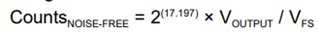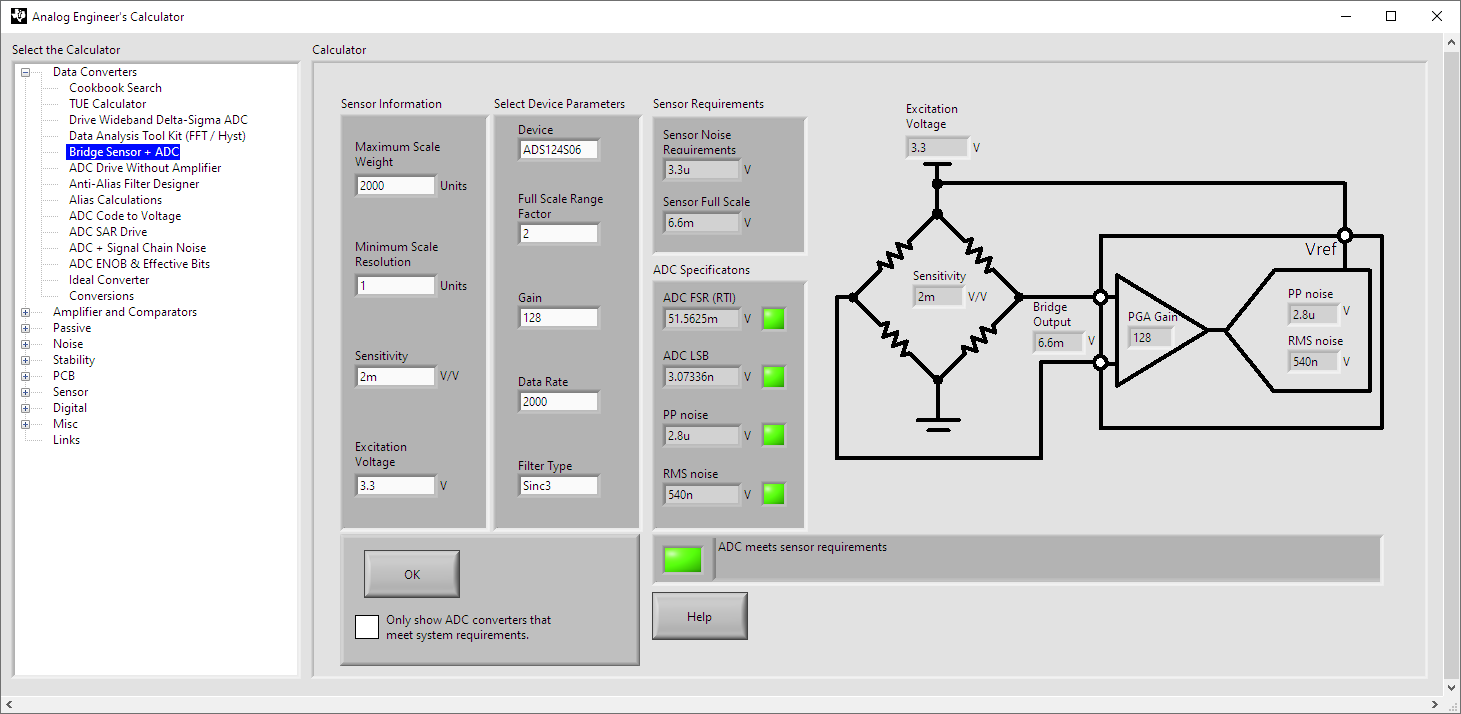If you have a related question, please click the "Ask a related question" button in the top right corner. The newly created question will be automatically linked to this question.

# ADS124S06: How to reduce noise for High sampling rates?

Hi there,

We have selected ADS124S06 for Bridge sensor.

Bridge sensor specifications:

1.   Excitation voltage : 3.3V

2.   Sensitivity : 2mV/V

3.   Capacity : 2Kg

Our required Programmable data rate is 2000kSPS.

By setting this, We are getting Peak-to-Peak noise as 2.8uV (By using Analog Engineer's calculator).

Using these data, We are getting only 14.168 Bits(Noise free bits) - 2357 Counts instead of 24 bits. By using this formula,Refer : ADS1x4S08 Evaluation Module User's Guide (Rev. A)

How to get maximum Effective number of bits (ENOB)? How to reduce noise for High sampling rates?

Kindly give your suggestions on this.

Thanks & Regards,

Sneha K

• Hi Sneha K,

There are a couple of things to consider.  First, the full-scale range (FSR) is equal to 2*Vref / GAIN, so if Vref is equal to the supply (3.3V) and gain is at 128, then the FSR is about 51.6mV.  The full-scale load cell output is sensitivity (2mV/V) times excitation voltage (3.3V) where the maximum output of the load cell is 6.6mV.  So right away the number of available codes (2^24) is reduced by the ratio of the maximum load cell output (6.6mV) divided by the FSR (51.6mV).  2^24 * 6.6 / 51.6 which is about 2145923 total available codes (21 bits) assuming a perfect noise-free solution.

If we consider the noise of the ADC we can use the lookup tables in the datasheet.  Here you need to choose a digital filter (sinc3 for this example) and data rate (2ksps) along with the PGA gain (128).  We see in table 1 of the datasheet that the noise will be 2.8uV P2P.  P2P is used as that is the value that becomes stable, or sometimes called flicker-free.  You can translate to the number of noise bits by finding the number of codes of deviation and converting to log2.  The LSB size (smallest measurable code) is the FSR / 2^24, or 51.6mV / 2^24 which is about 3.07nV.  The number of noise codes is 2.8uV / 3.07nV or 912 codes (log(912) / log(2)) or 9.8 bits of noise. So 24 - 9.8 results in total noise-free bits of 14.2 bits.

So if we determine that instead of 2^24 total bits is replaced by noise-free bits (14.2) we can recalculate the total counts available for the load cell as 6.6mV / 51.6mV * 2^14.2 (or 2407 counts).  For a 2kg load cell you can achieve slightly better than 1g resolution.

In the analog engineers calculator tool you can find a bridge sensor calculator as shown below:The calculator tools shows the best resolution the ADC can do.  To further understand this topic I would suggest reviewing A Basic Guide to Bridge Measurements.

So the numbers shown is the best you can do with the given data rate (2ksps), 3.3V supply/reference/excitation and gain of 128.  You can improve slightly by lowering the data output rate, using 5V analog supply or using an external amplifier to increase the dynamic range.  However, adding external components will also add amplifier noise and drift.

Best regards,

Bob B

• Hi Bob,

Thanks for your quick response. Clarified now.